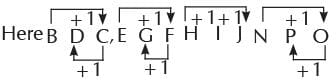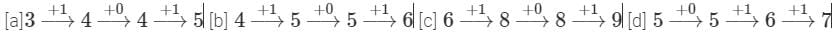# Olympiad Test: Series And Pattern - 1

## 10 Questions MCQ Test Mathematics Olympiad Class 4 | Olympiad Test: Series And Pattern - 1

Description
Attempt Olympiad Test: Series And Pattern - 1 | 10 questions in 20 minutes | Mock test for Class 4 preparation | Free important questions MCQ to study Mathematics Olympiad Class 4 for Class 4 Exam | Download free PDF with solutions
QUESTION: 1

Solution:
QUESTION: 2

### Which one is different from the others?

Solution:∴ HIJ is different.

QUESTION: 3

### Identify the one that does not belong to the group.

Solution:
QUESTION: 4

Identify the one which is different from others.

Solution:
QUESTION: 5

Find the odd one out.

Solution:

All options are a pair of consecutive three alphabets written in opposite order, except for option c

QUESTION: 6

Which one is different from the others?

Solution:

Sum of digits of each number is divisible by 3

QUESTION: 7

Identify the one which does not belong to the others.

Solution:
QUESTION: 8

Identify the one which is different from the others.

Solution:So D is correct option.

QUESTION: 9

Find the odd one out.

Solution:

75444 is a 5 digit number

QUESTION: 10

Which one is different from others?

Solution:Use Code STAYHOME200 and get INR 200 additional OFF Use Coupon Code